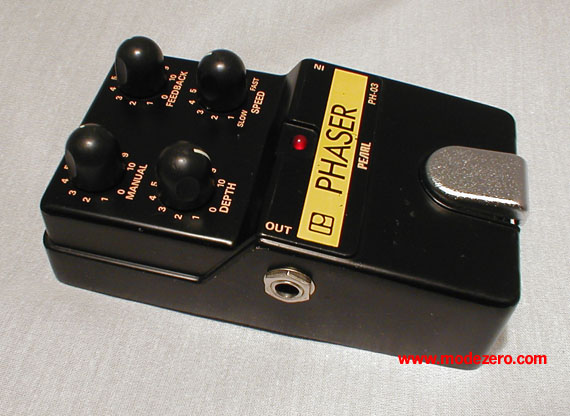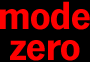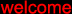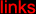(c) 2000-2009

mode zero

pearl ph-03 phaser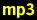guitar, no effectguitar, manual = 5, depth = 5, feedback = 5, speed = 7guitar, manual = 6, depth = 10, feedback = 0, speed = 3guitar, manual = 8, depth = 8, feedback = 9, speed = 2matrix-1000 pad, no effectmatrix-1000 pad, manual = 5, depth = 6, feedback = 0, speed = 8matrix-1000 pad, manual = 8, depth = 8, feedback = 9, speed = slowmatrix-1000 pad, manual = 0 --> 10, depth = 10, feedback = 10, speed = fast